﻿ A-110.6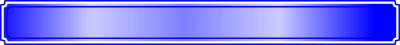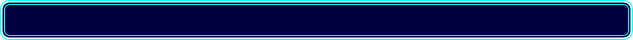Analogue  Modular  System  A-100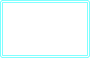WidthHPDepthmmCurrentmA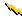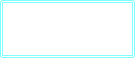Owners ManualNo manual available

12

55

10HP

80

10HP

A-110-6 is a Trapezoid Thru Zero Quadrature VCO based on a revised  idea by Donald Tillman. It’s unique trapezoid core is totally different compared to other VCOs, and it’s quadrature VCO features linear thru zero frequency modulation.  The term "quadrature" here means that the oscillator outputs two trapezoid waves with 90 degrees phase shift, similar to the A-110.4 or A-143.9.  These waveforms are TRASIN (trapezoid sine) and TRACOS (trapezoid cosine).
"Thru-Zero" means that "negative" frequencies are generated, so the TRASIN/TRACOS waves will stop when the linear CV reaches 0V and continues with the opposite direction as the CV rises/falls.
There are two control sections: linear and exponential. The exponential section consists of the XTune control, the 1V/Oct input and the XFM input with attenuator XFM. The exponential CV is the sum of these three voltages. The linear section consists of the LFrq control and the LFM input with attenuator LFM. The linear CV is the sum of these two voltages. A bi-colour LED shows the polarity of the linear CV. The pitch of all outputs is determined by the CV of both sections. The linear section is used to control the pitch in a linear manner. When the LFrq control is fully CW the module works like a normal VCO and the LED lights red. The pitch is then controlled by the exponential section with the manual Tune control XTune and the exponential frequency control inputs 1V/Oct and XFM. 1V/Oct is used to control the pitch, XFM is used to apply an exponential frequency modulation with adjustable depth. As the LFrq control is turned counterclockwise starting from the fully CW position the frequency is lowered in a linear manner until the TRASIN/TRACOS waves (nearly) stop at the centre position of LFrq (provided that no LFM signal is present). As the LFrq control is moved from the centre towards the CCW position the waves start again but into reverse direction and the LED turns yellow. When the fully CCW position of LFrq is reached the module works again like a normal VCO. But much more exciting is the usage of the LFM input to modify the linear control voltage by an external control voltage (typically another VCO). Linear modulation by another oscillator using the thru zero feature in combination with the trapezoid waveforms generates audio spectra than cannot be obtained from any other oscillator without the thru zero function.
Other waveforms like triangle, sawtooth, rectangle and sine are obtained from the TRASIN/TRACOS signals, all five waveforms are available as quadrature pairs (i.e. with 90 degrees phase shift).